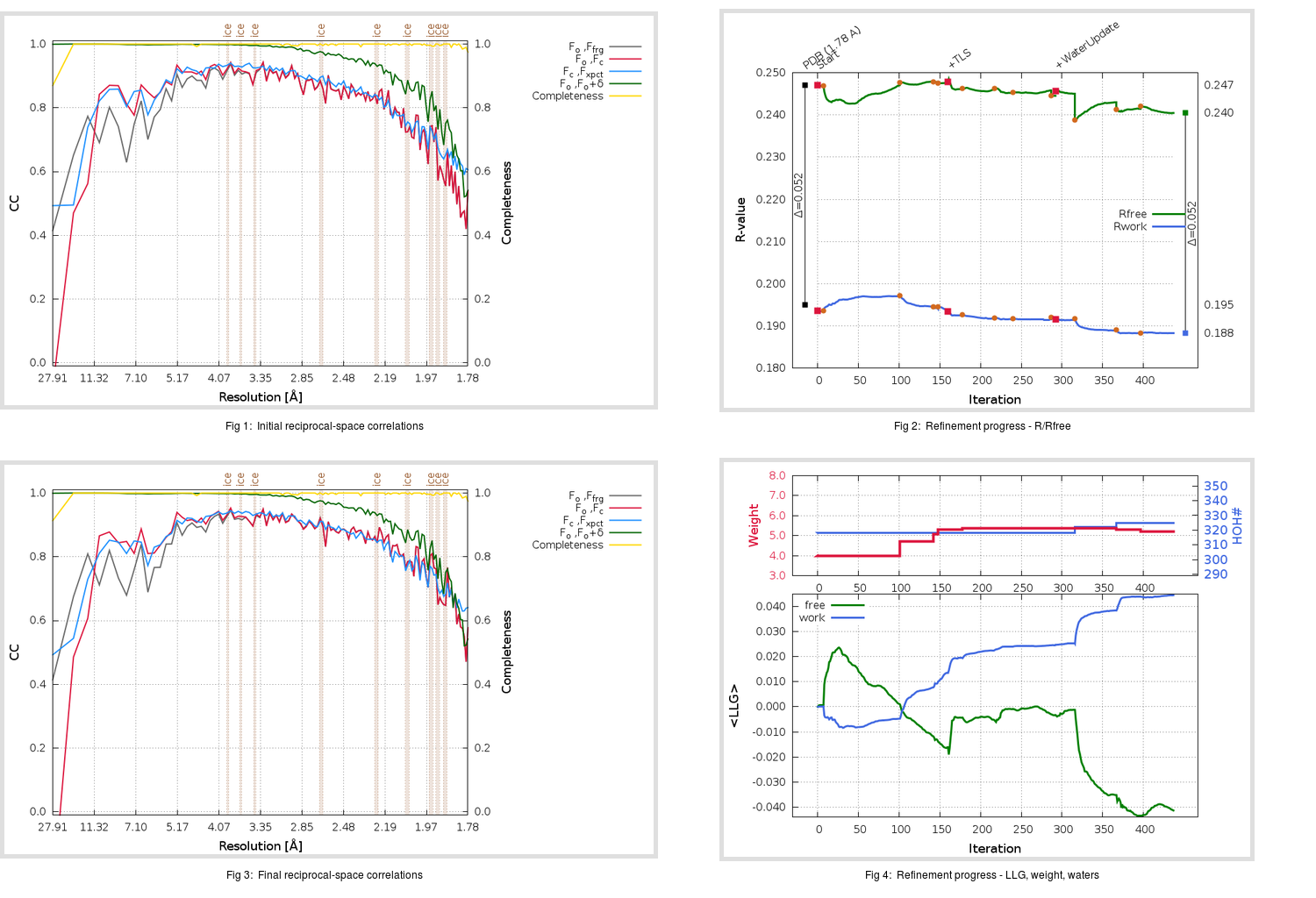Content:

## Deposited

` `
 Date deposited Date data collection Resolution R, Rfree 20200315 20200305 1.78 0.1920 0.2470

Molprobity (CCP4 7.0 version) summary:

```Ramachandran outliers =   0.66 %
favored =  97.35 %
Rotamer outliers      =   1.14 %
C-beta deviations     =     0
Clashscore            =   4.86
RMS(bonds)            =   0.0130
RMS(angles)           =   1.80
MolProbity score      =   1.42
Resolution            =   1.78
R-work                =   0.1920
R-free                =   0.2470
```

```Number of waters      =   318

<B> (all atoms) =   23.32 ( sd =    9.57 ) for       2719 non-hydrogen atoms
<B>   (protein) =   22.25 ( sd =    9.11 ) for       2370 non-hydrogen atoms
<B>     (water) =   31.06 ( sd =    9.42 ) for        318 non-hydrogen atoms
<B>    (others) =   29.38 ( sd =   13.36 ) for         31 non-hydrogen atoms

B min/max       (all non-hydrogen atoms) =    9.51 /   78.76
B min/max   (protein non-hydrogen atoms) =   11.96 /   78.76
B min/max     (water non-hydrogen atoms) =    9.51 /   57.22
B min/max     (other non-hydrogen atoms) =   19.20 /   40.43
```

## BUSTER (re-)refinement

` `

Molprobity (CCP4 7.0 version) summary:

```Ramachandran outliers =   0.33 %
favored =  98.34 %
Rotamer outliers      =   1.14 %
C-beta deviations     =     0
Clashscore            =   2.11
RMS(bonds)            =   0.0119
RMS(angles)           =   1.58
MolProbity score      =   1.03
Resolution            =   1.78
R-work                =   0.1883
R-free                =   0.2405
```

```Number of waters      =   325

<B> (all atoms) =   24.39 ( sd =    8.91 ) for       2726 non-hydrogen atoms
<B>   (protein) =   23.08 ( sd =    7.89 ) for       2370 non-hydrogen atoms
<B>     (water) =   33.56 ( sd =    9.98 ) for        325 non-hydrogen atoms
<B>    (others) =   31.38 ( sd =   15.57 ) for         31 non-hydrogen atoms

B min/max       (all non-hydrogen atoms) =    7.34 /   71.72
B min/max   (protein non-hydrogen atoms) =    8.37 /   71.72
B min/max     (water non-hydrogen atoms) =    7.34 /   61.99
B min/max     (other non-hydrogen atoms) =   19.69 /   48.56
```

Refinement progression:Results:

` `
 File Remark 5RFF_aB_refine.01_03_refine.pdb.gz exact refinement commands are in header 5RFF_aB_refine.01_03_refine.mtz.gz including original deposited data and several re-refinement map coefficients 5RFF_aB_refine.01_03_BUSTER_model.cif.gz including any non-standard compound restraints 5RFF_aB_refine.01_03_BUSTER_refln.cif.gz